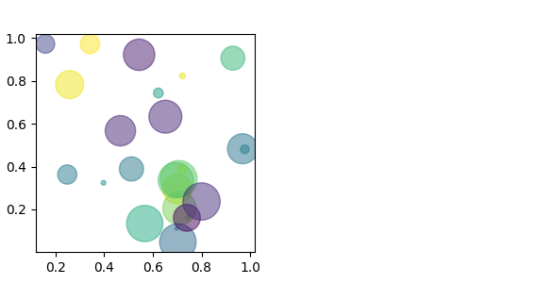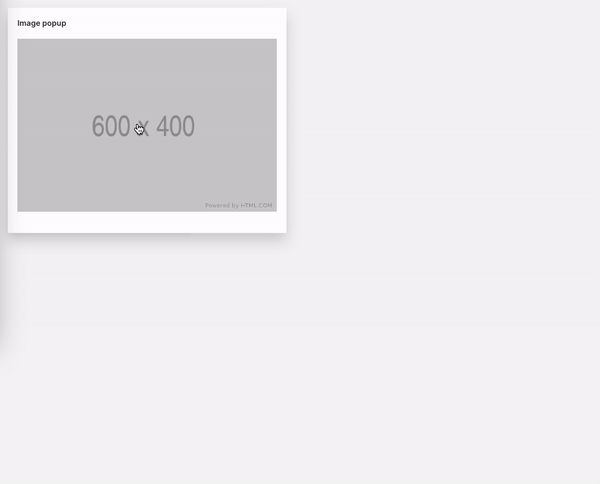# Image

Make your app come to life by including interesting imagery.

Check the full API at ui.image.

## Basic image​``path = 'https://raw.githubusercontent.com/h2oai/wave/main/assets/brand/wave-university-wide.png'q.page['example'] = ui.form_card(box='1 1 8 6', items=[    ui.image(title='Image title', path=path),])``

## Base64 image​

Wave also supports images in Base64 format. You need to provide `image` with a base64 string and `type` of the image.``import ioimport base64import numpy as npimport matplotlib.pyplot as pltnp.random.seed(19680801)n = 25plt.figure(figsize=(3, 3))plt.scatter(    np.random.rand(n), np.random.rand(n),    s=(30 * np.random.rand(n)) ** 2,    c=np.random.rand(n),    alpha=0.5,)buf = io.BytesIO()plt.savefig(buf, format='png')buf.seek(0)image = base64.b64encode(buf.read()).decode('utf-8')q.page['example'] = ui.form_card(box='1 1 4 4', items=[    ui.image(title='Image title', image=image, type='png'),])``

Another alternative how to make use of Base64 images is constructing a data URL.``import ioimport base64import numpy as npimport matplotlib.pyplot as pltnp.random.seed(19680801)n = 25plt.figure(figsize=(3, 3))plt.scatter(    np.random.rand(n), np.random.rand(n),    s=(30 * np.random.rand(n)) ** 2,    c=np.random.rand(n),    alpha=0.5,)buf = io.BytesIO()plt.savefig(buf, format='png')buf.seek(0)image = base64.b64encode(buf.read()).decode('utf-8')q.page['example'] = ui.form_card(box='1 1 4 4', items=[    ui.image(title='Image title', path=f'data:image/png;base64,{image}'),])``

## Markdown image​

Apart from using a dedicated ui.image component, one can also include images using any textual component that supports Markdown. This way requires regular image URLs (no base64).``content = '![Wave University](https://raw.githubusercontent.com/h2oai/wave/main/assets/brand/wave-university-wide.png)'q.page['example'] = ui.form_card(box='1 1 8 6', items=[    ui.text(content=content)])``

## Image popup​

Displaying images comes with an inherent performance cost due to their file size. Wouldn't it be nice if you could display a smaller image on the initial render to give your users a "preview" and if it catches their attention, allow them to click the image and show it in its full size throughout the whole screen? The good news is you can thanks to a `path_popup` attribute that accepts a path or a URL or a data URL of the high-resolution version of your image. Note, that this does not replace a `path` property.``q.page['example'] = ui.form_card(box='1 1 3 4', items=[    ui.image(        title='Image popup',         path='https://via.placeholder.com/600x400',         path_popup='https://via.placeholder.com/1200x800'    ),])``